# RD Sharma Solutions Class 9 Maths Chapter 23 Graphical Representation Of Statistical Data Exercise 23.2

In this exercise, students will learn about bar graphs and their constructions. A bar graph is a pictorial representation of the numerical data by a number of bars of uniform width erected vertically or horizontally with equal spacing between them. The bar graph is one of the easiest ways to understand and analyze big data or information. RD Sharma Solutions Class 9 Chapter 23 textbook questions and answers helps students to understand the concept in an easy way.

## Download PDF of RD Sharma Solutions for Class 9 Maths Chapter 23 Graphical Representation of Statistical Data Exercise 23.2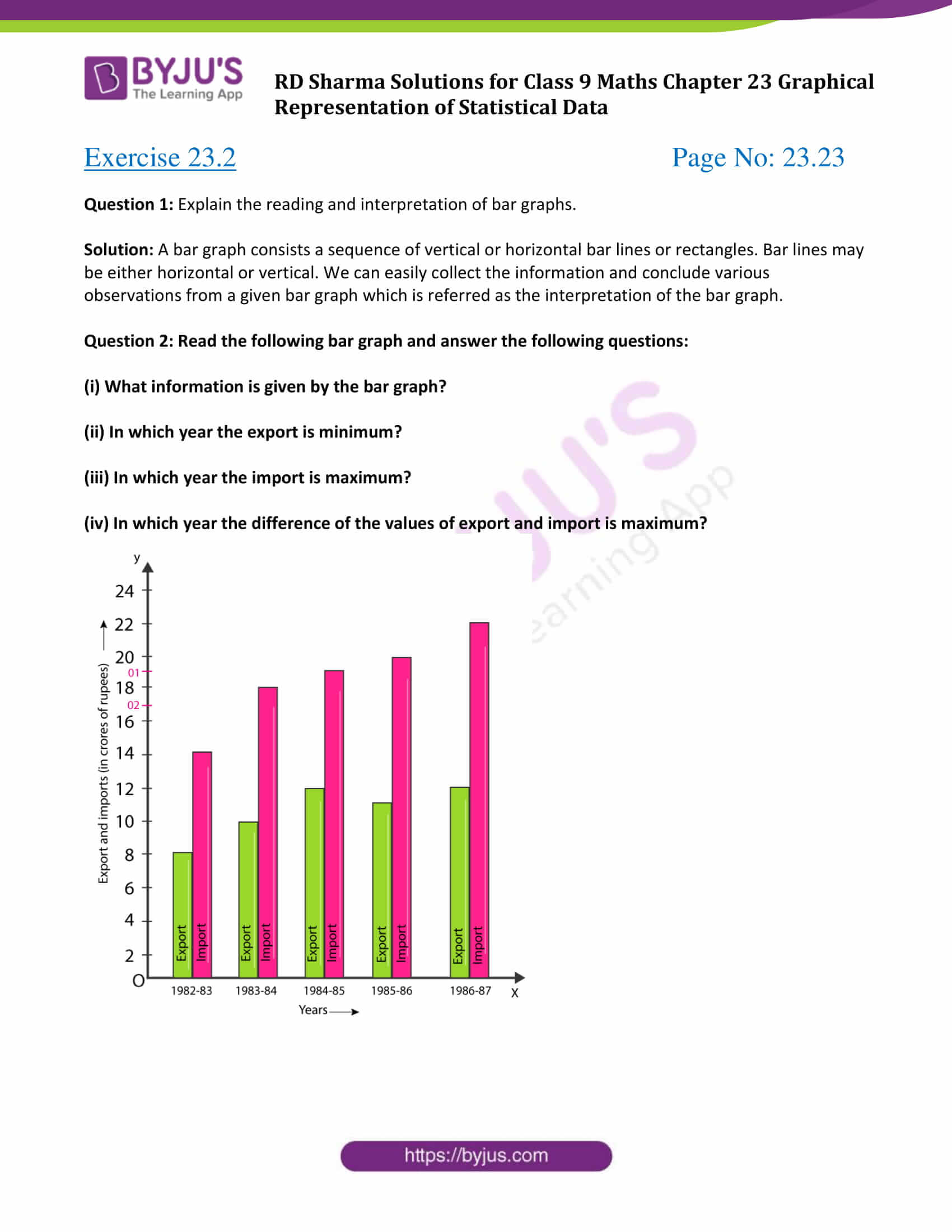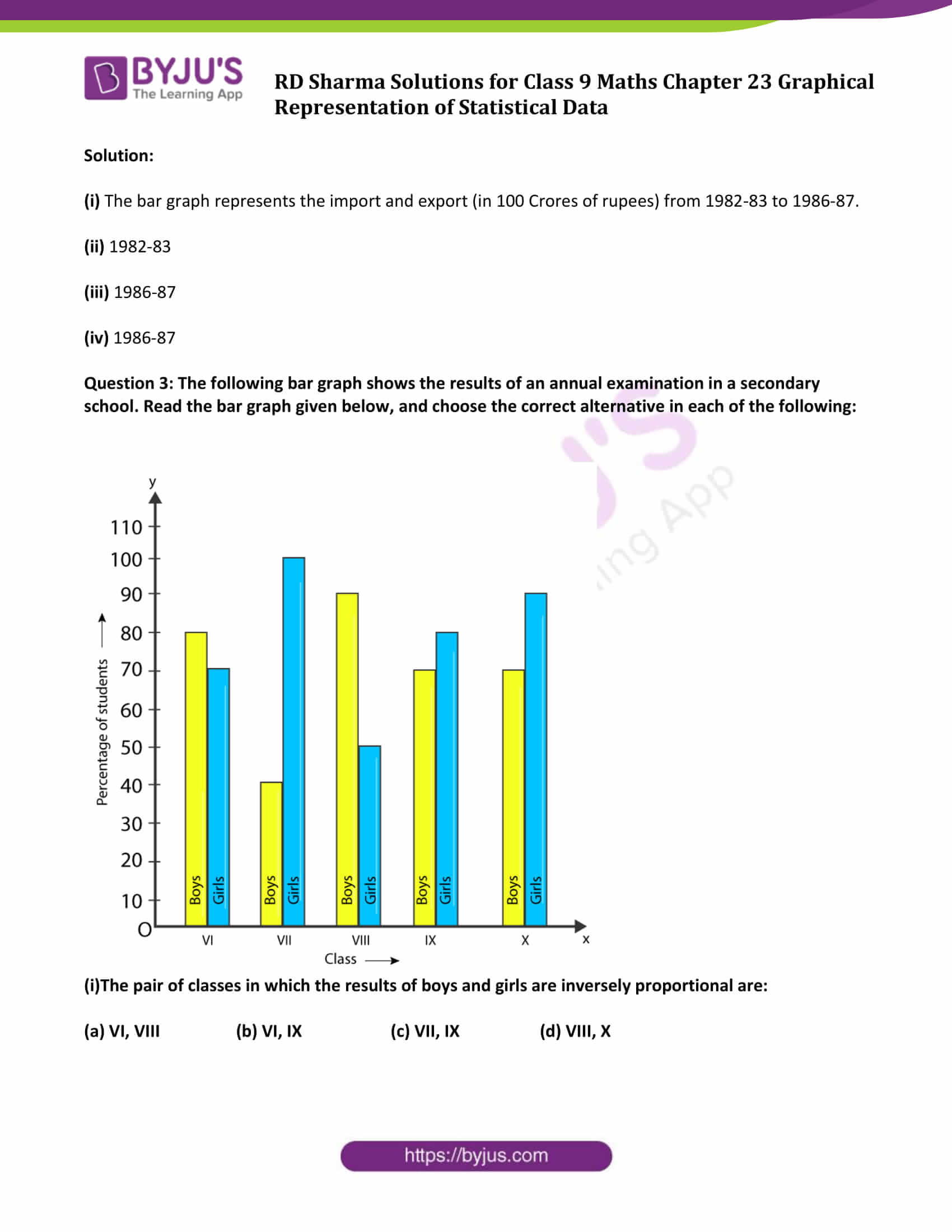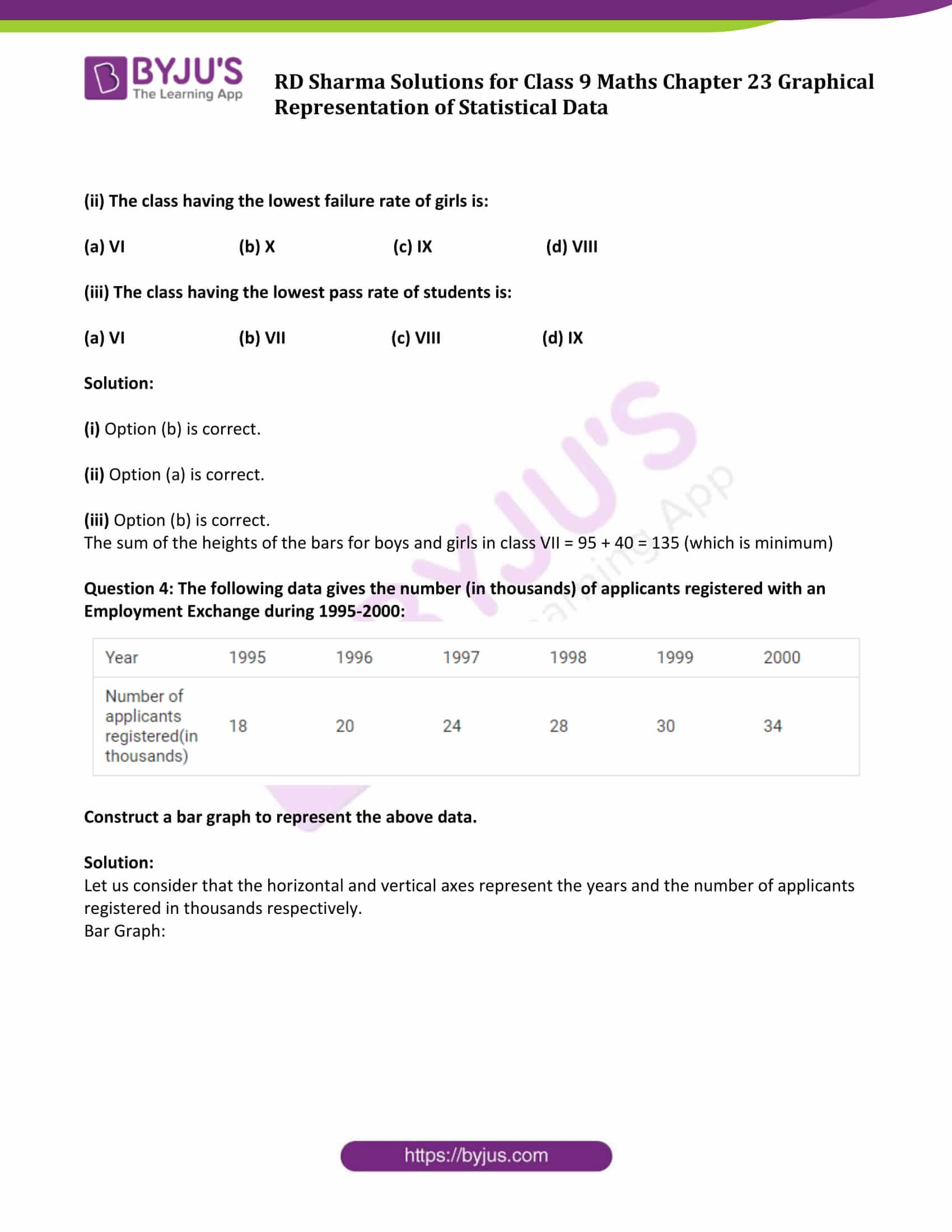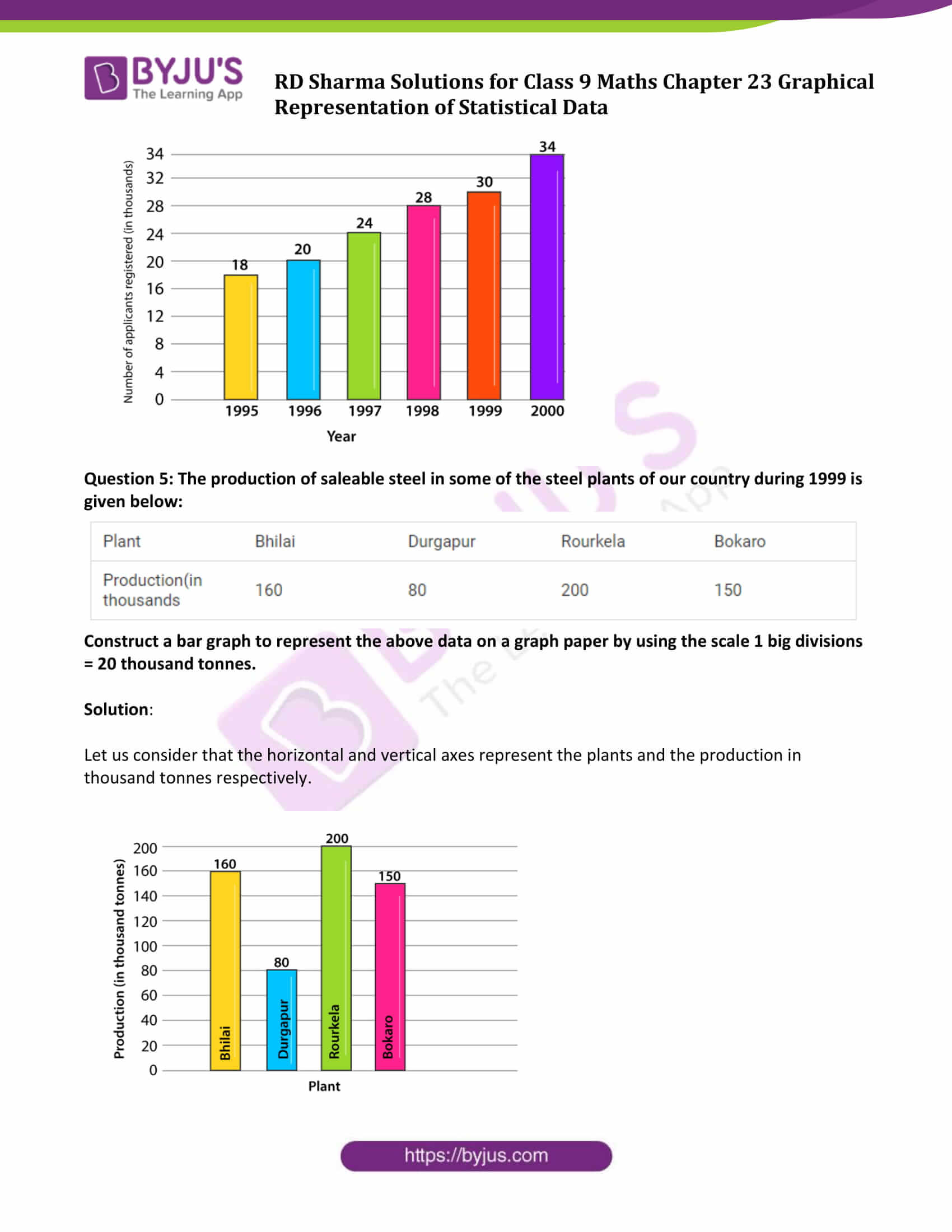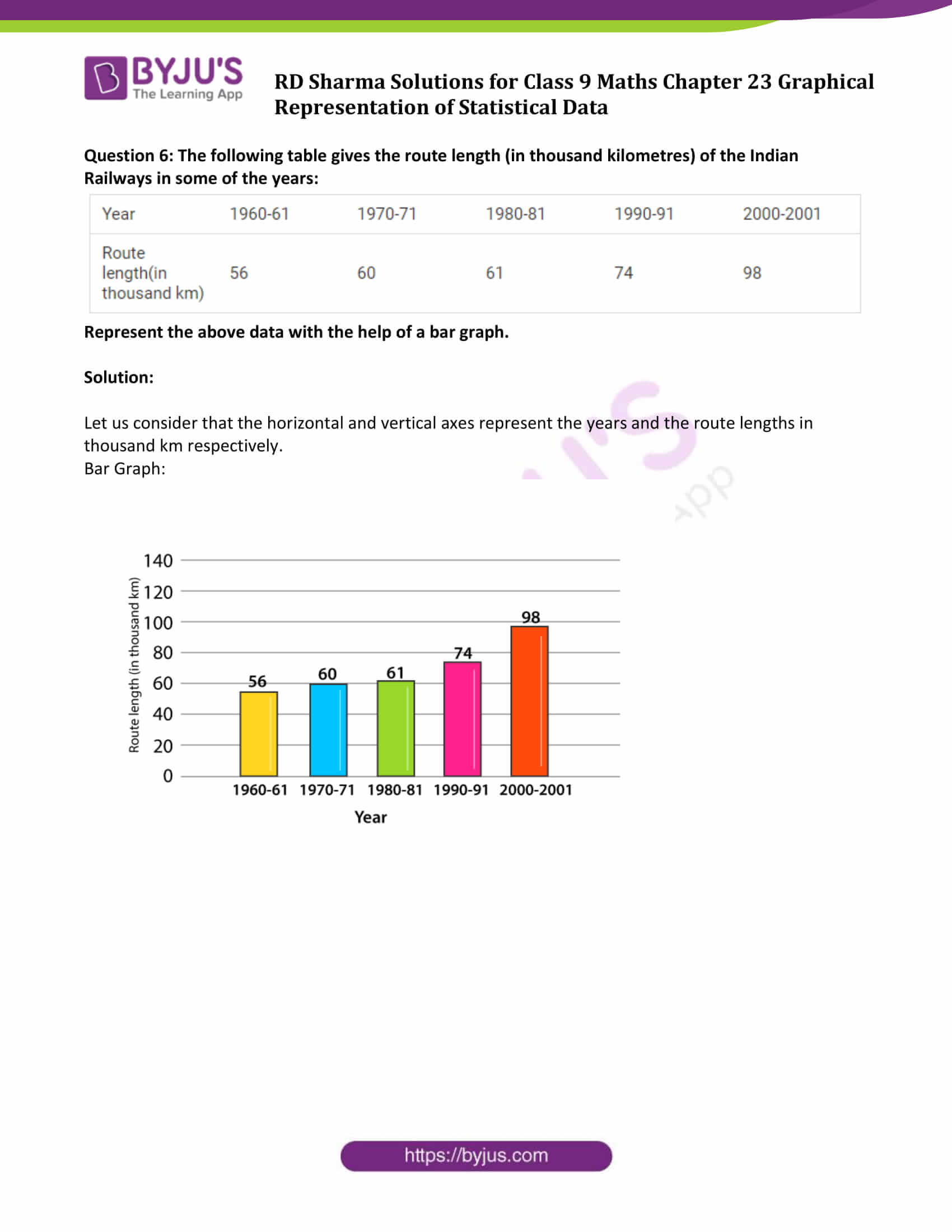### Access Answers to Maths RD Sharma Solutions for Class 9 Chapter 23 Graphical Representation of Statistical Data Exercise 23.2 Page number 23.7

Question 1: Explain the reading and interpretation of bar graphs.

Solution: A bar graph consists a sequence of vertical or horizontal bar lines or rectangles. Bar lines may be either horizontal or vertical. We can easily collect the information and conclude various observations from a given bar graph which is referred as the interpretation of the bar graph.

Question 2: Read the following bar graph and answer the following questions:

(i) What information is given by the bar graph?

(ii) In which year the export is minimum?

(iii) In which year the import is maximum?

(iv) In which year the difference of the values of export and import is maximum?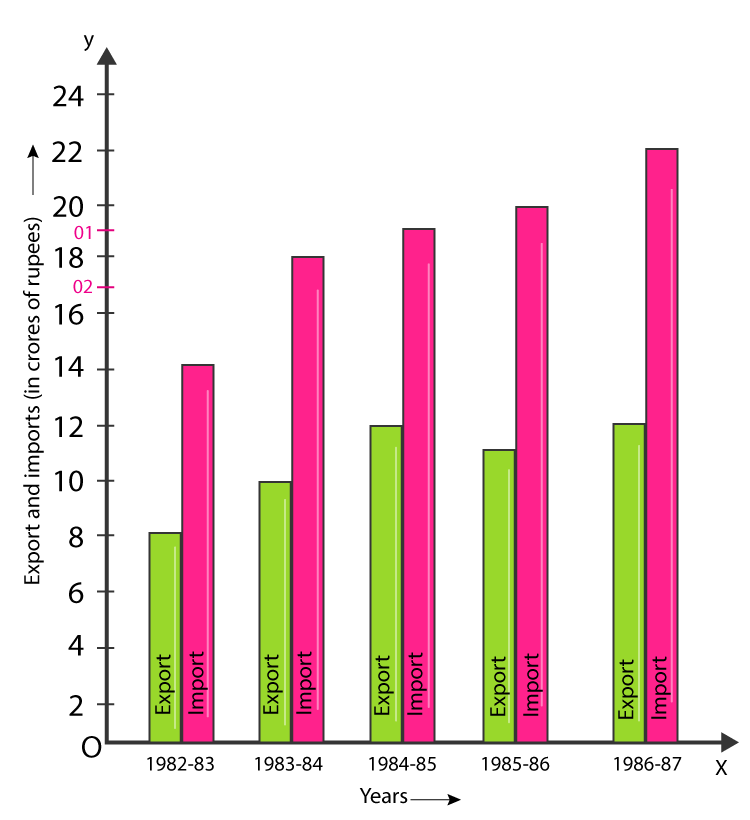Solution:

(i) The bar graph represents the import and export (in 100 Crores of rupees) from 1982-83 to 1986-87.

(ii) 1982-83

(iii) 1986-87

(iv) 1986-87

Question 3: The following bar graph shows the results of an annual examination in a secondary school. Read the bar graph given below, and choose the correct alternative in each of the following: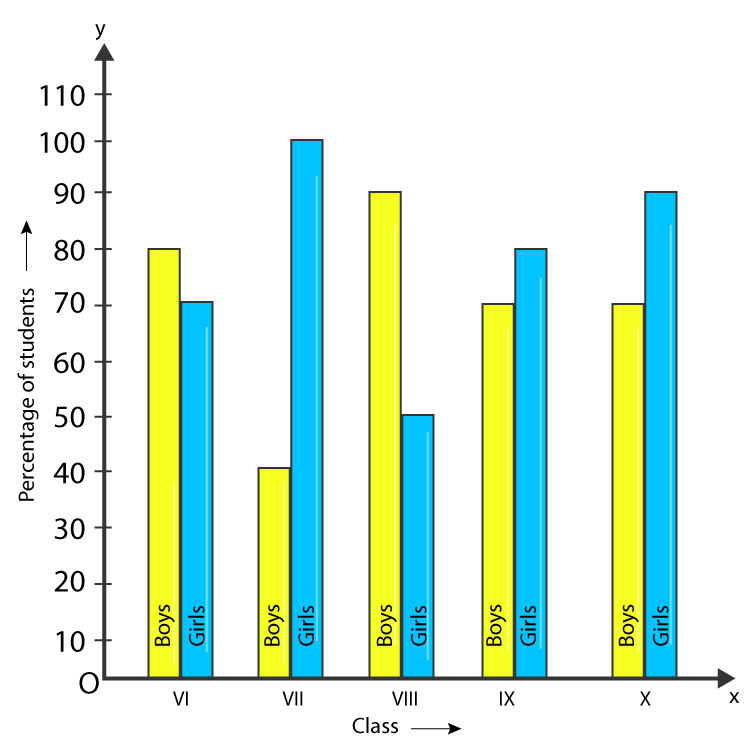(i)The pair of classes in which the results of boys and girls are inversely proportional are:

(a) VI, VIII (b) VI, IX (c) VII, IX (d) VIII, X

(ii) The class having the lowest failure rate of girls is:

(a) VI (b) X (c) IX (d) VIII

(iii) The class having the lowest pass rate of students is:

(a) VI (b) VII (c) VIII (d) IX

Solution:

(i) Option (b) is correct.

(ii) Option (a) is correct.

(iii) Option (b) is correct.

The sum of the heights of the bars for boys and girls in class VII = 95 + 40 = 135 (which is minimum)

Question 4: The following data gives the number (in thousands) of applicants registered with an Employment Exchange during 1995-2000: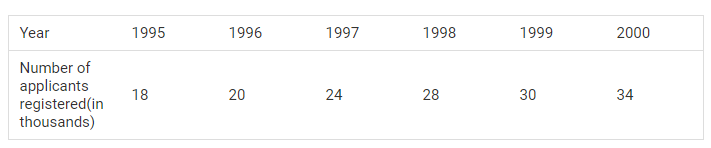Construct a bar graph to represent the above data.

Solution:

Let us consider that the horizontal and vertical axes represent the years and the number of applicants registered in thousands respectively.

Bar Graph: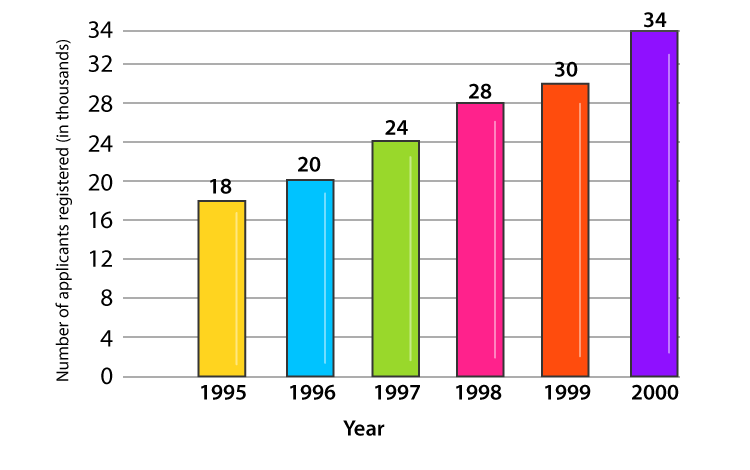Question 5: The production of saleable steel in some of the steel plants of our country during 1999 is given below: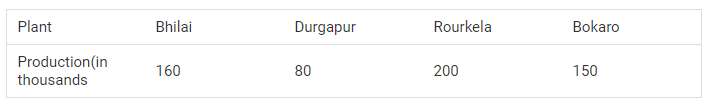Construct a bar graph to represent the above data on a graph paper by using the scale 1 big divisions = 20 thousand tonnes.

Solution:

Let us consider that the horizontal and vertical axes represent the plants and the production in thousand tonnes respectively.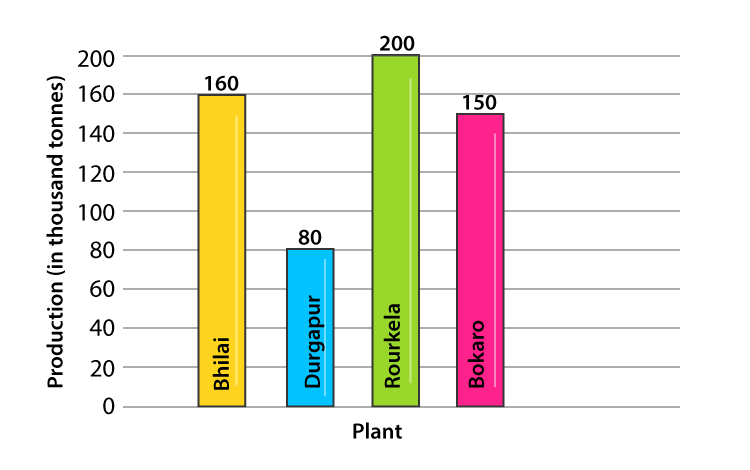Question 6: The following table gives the route length (in thousand kilometres) of the Indian Railways in some of the years:Represent the above data with the help of a bar graph.

Solution:

Let us consider that the horizontal and vertical axes represent the years and the route lengths in thousand km respectively.

Bar Graph: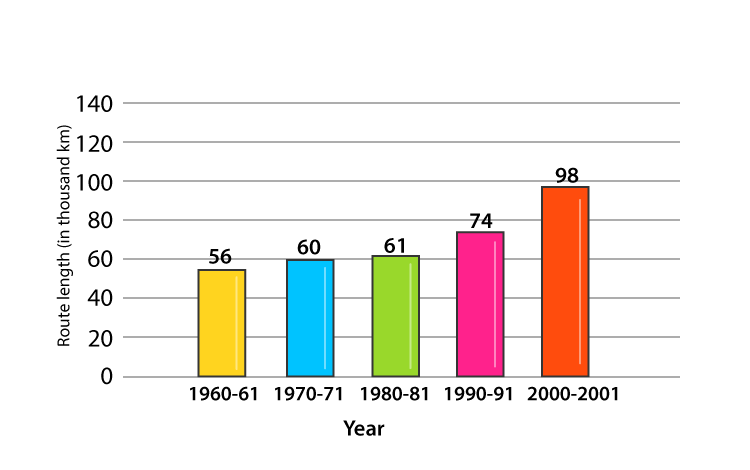## RD Sharma Solutions for Class 9 Maths Chapter 23 Graphical Representation of Statistical Data Exercise 23.2

RD Sharma Solutions Class 9 Maths Chapter 23 Graphical Representation of Statistical Data Exercise 23.2 is based on the topic – Construction of bar graphs.

Students can understand this concept in detail with complete exercise wise solved RD Sharma Solutions to make your learning easier and prepare well for understanding different graphical problems quickly.